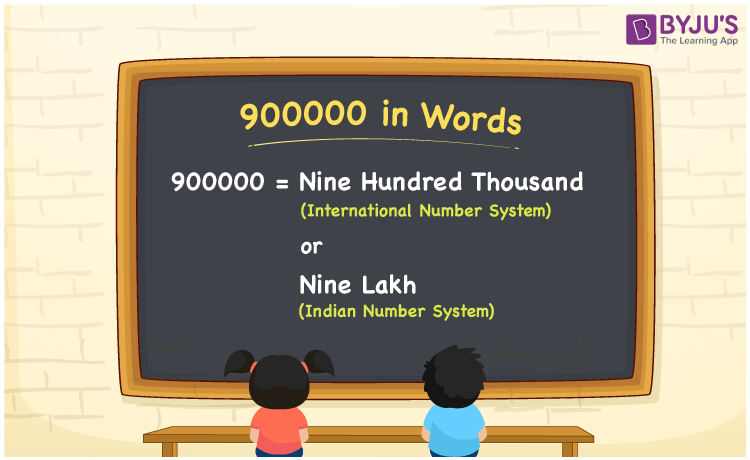# 900000 in Words

We can write 900000 in words as Nine hundred thousand in the International number system and as Nine lakh in the Indian number system. In this article, you will learn how to convert the cardinal number 900000 to words of both International and Indian number systems.

 900000 in words Nine hundred thousand  (or) Nine lakh Nine hundred thousand  (or) Nine lakh in Numbers 900000

## 900000 in English Words

We generally write numbers in words using the English alphabet. Thus, we can read 900000 in English as “Nine lakh”.## How to Write 900000 in Words?

900000 in words – International number system

The below table depicts the place value chart for 900000 with respect to the International number system.

 Thousands Hundreds Tens Ones 900 0 0 0

Here, ones = 0, tens = 0, hundreds = 0, thousands = 250

Therefore, 900000 in words = 900 thousand = Nine hundred thousand

900000 in words – Indian number system

Given below is the place value chart for 900000 in the Indian system.

 Lakhs Ten thousands Thousands Hundreds Tens Ones 9 0 0 0 0 0

Here, ones = 0, tens = 0, hundreds = 0, thousands = 0, ten thousands = 0 lakhs = 9

That means, 9 lakhs

= Nine lakh

900000 is a natural number that precedes 900001 and succeeds 899999.

900000 in words – Nine hundred thousand (or) Nine lakh

Is 900000 an odd number? – No

Is 900000 an even number? – Yes

Is 900000 a perfect square number? – No

Is 900000 a perfect cube number? – No

Is 900000 a prime number? – No

Is 900000 a composite number? – Yes

## Frequently Asked Questions on 900000 in Words

Q1

### How do you say 900000 in words?

We can say 900000 in words as Nine hundred thousand (or) Nine lakh.
Q2

### How do you write Rs. 900000 in words for a cheque?

For a cheque, we write Rs. 90000 in words as “Nine lakh rupees only”.
Q3

### Write the answer in words for 800000 + 100000.

800000 + 100000 = 900000
Therefore, the value of 800000 + 100000 in words is Nine hundred thousand (or) Nine lakh.